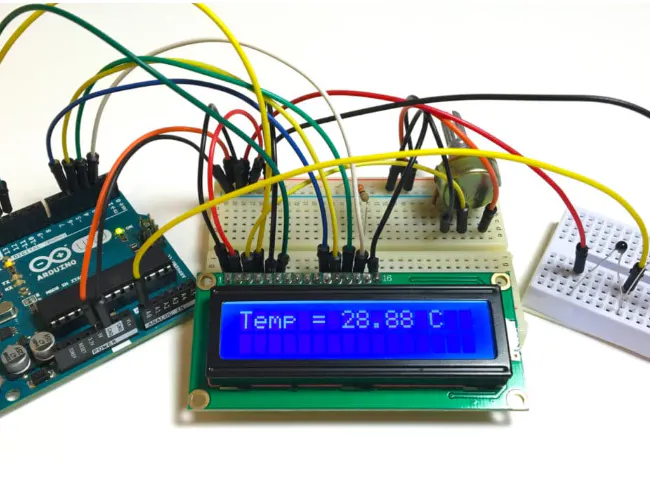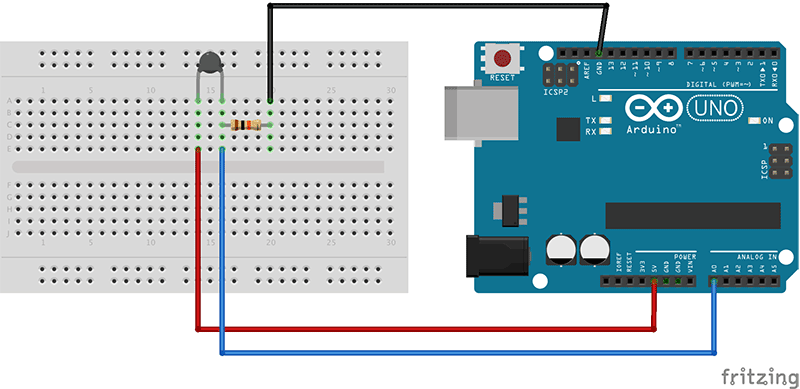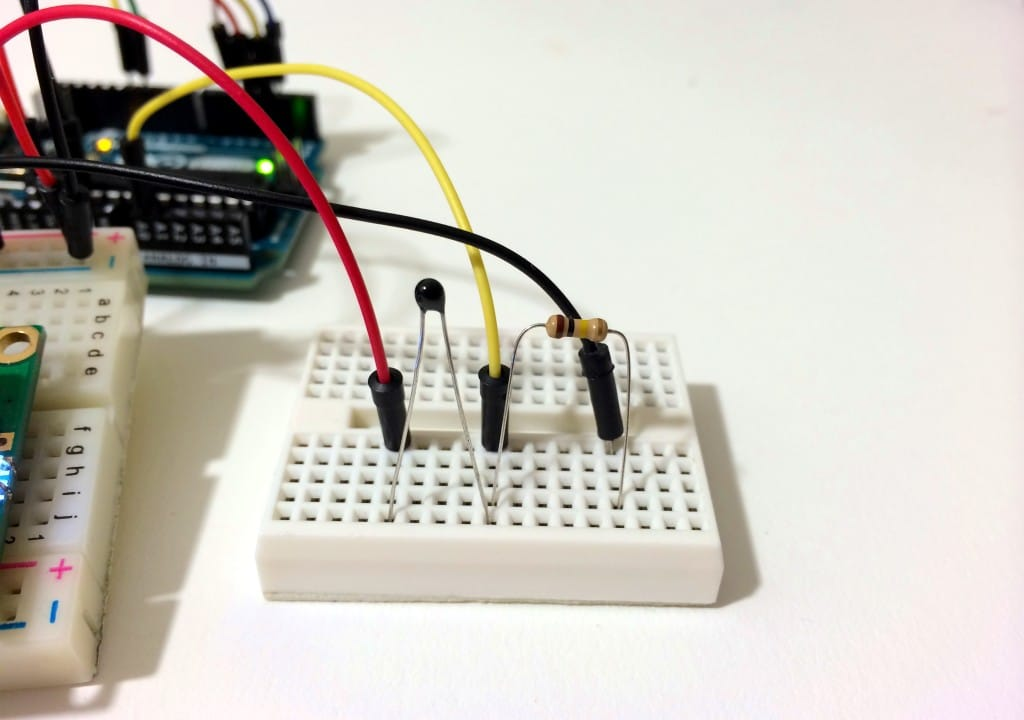Project tutorial# Make an Arduino Temperature Sensor (thermistor tutorial) © LGPL

Thermistors are simple, inexpensive, and accurate components that make it easy to get temperature data for your projects.

• 64,402 views
• 11 comments
• 35 respects

## About this project

### A THERMISTOR WORKS

Thermistors are variable resistors that change their resistance with temperature. They are classified by the way their resistance responds to temperature changes. In Negative Temperature Coefficient (NTC) thermistors, resistance decreases with an increase in temperature. In Positive Temperature Coefficient (PTC) thermistors, resistance increases with an increase in temperature.

NTC thermistors are the most common, and that’s the type we’ll be using in this tutorial. NTC thermistors are made from a semiconducting material (such as a metal oxide or ceramic) that’s been heated and compressed to form a temperature sensitive conducting material.

The conducting material contains charge carriers that allow current to flow through it. High temperatures cause the semiconducting material to release more charge carriers. In NTC thermistors made from ferric oxide, electrons are the charge carriers. In nickel oxide NTC thermistors, the charge carriers are electron holes.

Let’s build a basic thermistor circuit to see how it works, so you can apply it to other projects later.

Since the thermistor is a variable resistor, we’ll need to measure the resistance before we can calculate the temperature. However, the Arduino can’t measure resistance directly, it can only measure voltage.

The Arduino will measure the voltage at a point between the thermistor and a known resistor. This is known as a voltage divider. The equation for a voltage divider is:

In terms of the voltage divider in a thermistor circuit, the variables in the equation above are:

This equation can be rearranged and simplified to solve for R2, the resistance of the thermistor:

Finally, the Steinhart-Hart equation is used to convert the resistance of the thermistor to a temperature reading.

The value of the resistor should be roughly equal to the resistance of your thermistor. In this case, the resistance of my thermistor is 100K Ohms, so my resistor is also 100K Ohms.

The manufacturer of the thermistor might tell you it’s resistance, but if not, you can use a multimeter to find out. If you don’t have a multimeter, you can make an Ohm meter with your Arduino by following our Arduino Ohm Meter tutorial. You only need to know the magnitude of your thermistor. For example, if your thermistor resistance is 34, 000 Ohms, it is a 10K thermistor. If it’s 340, 000 Ohms, it’s a 100K thermsitor.

## Code

##### CODE FOR SERIAL MONITOR OUTPUT OF TEMPERATURE READINGS:C/C++
After connecting the circuit above, upload this code to your Arduino to output the temperature readings to the serial monitor in Fahrenheit:
```int ThermistorPin = 0;
int Vo;
float R1 = 10000;
float logR2, R2, T;
float c1 = 1.009249522e-03, c2 = 2.378405444e-04, c3 = 2.019202697e-07;

void setup() {
Serial.begin(9600);
}

void loop() {

Vo = analogRead(ThermistorPin);
R2 = R1 * (1023.0 / (float)Vo - 1.0);
logR2 = log(R2);
T = (1.0 / (c1 + c2*logR2 + c3*logR2*logR2*logR2));
T = T - 273.15;
T = (T * 9.0)/ 5.0 + 32.0;

Serial.print("Temperature: ");
Serial.print(T);
Serial.println(" F");

delay(500);
}
```
##### CODE FOR LCD OUTPUT OF TEMPERATURE READINGSC/C++
To output the temperature readings to a 16X2 LCD.
```#include <LiquidCrystal.h>

int ThermistorPin = 0;
int Vo;
float R1 = 10000;
float logR2, R2, T;
float c1 = 1.009249522e-03, c2 = 2.378405444e-04, c3 = 2.019202697e-07;

LiquidCrystal lcd(12, 11, 5, 4, 3, 2);

void setup() {
Serial.begin(9600);
}

void loop() {

Vo = analogRead(ThermistorPin);
R2 = R1 * (1023.0 / (float)Vo - 1.0);
logR2 = log(R2);
T = (1.0 / (c1 + c2*logR2 + c3*logR2*logR2*logR2));
T = T - 273.15;
T = (T * 9.0)/ 5.0 + 32.0;

lcd.print("Temp = ");
lcd.print(T);
lcd.print(" F");

delay(500);
lcd.clear();
}
```
##### CODE FOR CELCIUS AND FAHRENHEITC/C++
This program will display Celsius and Fahrenheit at the same time:
```int ThermistorPin = 0;
int Vo;
float R1 = 10000;
float logR2, R2, T, Tc, Tf;
float c1 = 1.009249522e-03, c2 = 2.378405444e-04, c3 = 2.019202697e-07;

void setup() {
Serial.begin(9600);
}

void loop() {

Vo = analogRead(ThermistorPin);
R2 = R1 * (1023.0 / (float)Vo - 1.0);
logR2 = log(R2);
T = (1.0 / (c1 + c2*logR2 + c3*logR2*logR2*logR2));
Tc = T - 273.15;
Tf = (Tc * 9.0)/ 5.0 + 32.0;

Serial.print("Temperature: ");
Serial.print(Tf);
Serial.print(" F; ");
Serial.print(Tc);
Serial.println(" C");

delay(500);
}
```

## Custom parts and enclosures

Connect the thermistor and resistor to your Arduino like this:## Schematics## Comments

#### Ultrasonic Sensor with Alarm, LCD and Temperature

Project tutorial by MichDragstar

• 26,521 views
• 10 comments
• 36 respects

#### DHT11 Humidity + Temperature Sensor with 16x2 LCD display

Project showcase by onatto22

• 53,183 views
• 13 comments
• 58 respects

• 77,718 views
• 18 comments
• 44 respects

#### Temperature sensor

Project tutorial by Grant

• 53,098 views
• 13 comments
• 57 respects

#### Room temperature sensor with display on Mobile

Project showcase by Kaustubh Agarwal

• 37,809 views
• 9 comments
• 68 respects

#### How To Use DS18B20 Water Proof Temperature Sensor

Project showcase by IoTBoys

• 152,678 views
• 25 comments
• 36 respects